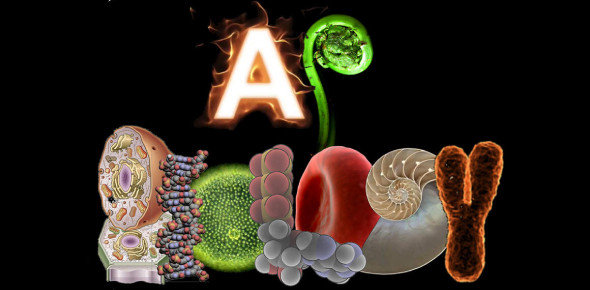# A.P. Biology Chapter 2 (Chemistry Review)

35 Questions | Total Attempts: 5141SettingsThis quiz is to test your knowledge on Chapter 2 of A. P. Biology. Chapter 2 will be on Test #1, which fill feature Chapters 1-4. As such, it is suggested that you also take the quizzes for Chapters 1, 3, and 4 if you are using this to study. To begin, type your name in the box below and click the green button.

• 1.
What are elements?
• A.

The simplest category of matter

• B.

The result of combining 2 compounds

• C.

Components which make up an atom

• D.

None of the above

• 2.
How many elements occur in nature?
• A.

25

• B.

24

• C.

92

• D.

91

• 3.
How many elements occur in life?
• A.

25

• B.

24

• C.

92

• D.

91

• 4.
• A.

0%

• B.

• C.

~4%

• D.

96%

• 5.
Name the 4 macroelements
• A.

Carbon, Oxygen, Sodium, Chlorine

• B.

Calcium, Potassium, Sodium, Chlorine

• C.

Carbon, Oxygen, Hydrogen, Nitrogen

• D.

• 6.
• A.

0%

• B.

• C.

~4%

• D.

96%

• 7.
Name the 6 microelements
• A.

Calcium, Potassium, Phosphorus, Sulfur, Sodium, Chlorine

• B.

Chromium, Cobalt, Copper, Flouride, Iodine, Iron

• C.

Carbon, Oxygen, Hydrogen, Nitrogen Sodium, Chlorine

• D.

Calcium, Potassium, Phosphorus, Sulfur, Iodine, Iron

• 8.
• A.

0%

• B.

• C.

~4%

• D.

96%

• 9.
Which of the following is a trace element?
• A.

Iron

• B.

Iodine

• C.

Zinc

• D.

All of the Above

• 10.
What is the atomic number?
• A.

# of protons in an atom

• B.

# of electrons in an atom, if not an ion

• C.

# of protons + # of neutrons

• D.

Both a and b

• 11.
What is the atomic mass?
• A.

# of protons in an atom

• B.

# of electrons in an atom, if not an ion

• C.

# of protons + # of neutrons

• D.

Both a and b

• 12.
How do you find the number of neutrons in an atom?
• A.

Atomic Number - Atomic Mass

• B.

# of protons - # of electrons

• C.

Atomic Mass - Atomic Number

• D.

# of electrons - # of protons

• 13.
What are isotopes?
• A.

Atoms with different numbers of neutrons

• B.

Atoms with different numbers of protons

• C.

Atoms with different numbers of electrons

• D.

None of the above

• 14.
What do you call an unstable isotope?
• A.

• B.

• C.

Both A and B

• D.

None of the above

• 15.
What is the halflife of an isotope?
• A.

How long it takes half of the radioisotope to destroy itself

• B.

How long it takes half of the radioisotope to repair itself

• C.

How long it takes half of the radioisotope gain neutrons

• D.

How long it takes half of the radioisotope to lose neutrons

• 16.
What is the halflife of Carbon 14? (in years)
• A.

50

• B.

500

• C.

5,000

• D.

50,000

• 17.
When will the amount of grams of an isotope hit zero?
• A.

In 5,000 years

• B.

In 10,000 years

• C.

In 20,000 years

• D.

Never

• 18.
• A.

To date fossils, to power cars, and to scan computers for viruses

• B.

To date fossils, to trace things, and to treat cancer

• C.

To treat cancer, to treat viruses, and to trace things

• D.

To date fossils, to power cars, and to treat cancer

• 19.
• A.

The energy is absorbed by electrons, which speed up

• B.

The energy is absorbed by electrons, which speed up and move to an outer energy level

• C.

The energy is absorbed by electrons, which speed up and move to an outer energy level, but later fall back into the original energy level

• D.

The energy is absorbed by electrons, which then speed up and move to an outer energy level, but later fall back to original energy level and give off energy in the form of light or passing it to another atom

• 20.
What are valence electrons?
• A.

The outermost electrons

• B.

The innermost electrons

• C.

Electrons that are involved in chemical reactions

• D.

Both A and C

• E.

Zoop Zop Zippity Bop

• 21.
How many electrons do stable elements have?
• A.

6

• B.

7

• C.

8

• D.

9

• 22.
Which elements have 8 valence electrons?
• A.

Halogens

• B.

Noble/inert gases

• C.

Earth Metals

• D.

Metalloids

• 23.
What will an atom if it lacks 8 valence electrons?
• A.

Seek to obtain 8 by bonding

• B.

Nothing

• C.

Seek to obtain 8 by creating more

• D.

Take electrons from lower energy levels to simulate having 8 in the outermost energy level

• 24.
What is covalent bonding?
• A.

Bonds in which 1 metal and 1 nonmetal will share electrons

• B.

Bonds in which 2 nonmetals will share electrons

• C.

Bonds in which 1 metal and 1 nonmetal will gain/lose electrons

• D.

Bonds in which 2 nonmetals will gain/lose electrons

• 25.
If there is a covalent bond in which 2 pairs of electrons are EQUALLY shared, that bond is...
• A.

Polar single

• B.

Polar double

• C.

Non-polar single

• D.

Non-polar double

Related TopicsBack to top